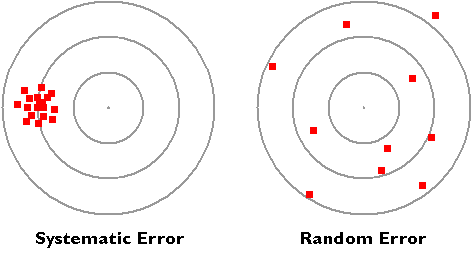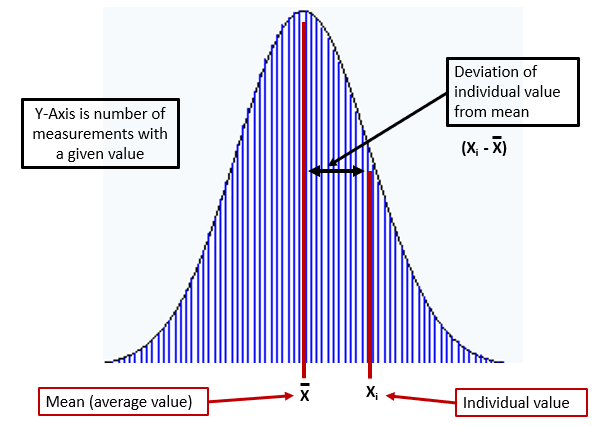# 1B.2: Making Measurements: Experimental Error, Accuracy, Precision, Standard Deviation and Significant Figures

$$\newcommand{\vecs}{\overset { \rightharpoonup} {\mathbf{#1}} }$$ $$\newcommand{\vecd}{\overset{-\!-\!\rightharpoonup}{\vphantom{a}\smash {#1}}}$$$$\newcommand{\id}{\mathrm{id}}$$ $$\newcommand{\Span}{\mathrm{span}}$$ $$\newcommand{\kernel}{\mathrm{null}\,}$$ $$\newcommand{\range}{\mathrm{range}\,}$$ $$\newcommand{\RealPart}{\mathrm{Re}}$$ $$\newcommand{\ImaginaryPart}{\mathrm{Im}}$$ $$\newcommand{\Argument}{\mathrm{Arg}}$$ $$\newcommand{\norm}{\| #1 \|}$$ $$\newcommand{\inner}{\langle #1, #2 \rangle}$$ $$\newcommand{\Span}{\mathrm{span}}$$ $$\newcommand{\id}{\mathrm{id}}$$ $$\newcommand{\Span}{\mathrm{span}}$$ $$\newcommand{\kernel}{\mathrm{null}\,}$$ $$\newcommand{\range}{\mathrm{range}\,}$$ $$\newcommand{\RealPart}{\mathrm{Re}}$$ $$\newcommand{\ImaginaryPart}{\mathrm{Im}}$$ $$\newcommand{\Argument}{\mathrm{Arg}}$$ $$\newcommand{\norm}{\| #1 \|}$$ $$\newcommand{\inner}{\langle #1, #2 \rangle}$$ $$\newcommand{\Span}{\mathrm{span}}$$$$\newcommand{\AA}{\unicode[.8,0]{x212B}}$$

Learning Objectives

• Differentiate between precision and accuracy
• Explain what significant Figures are
• Know and apply the rules of significant Figures to measured numbers

## Experimental Error

What is the difference between random and systematic error? There are two concepts we need to understand in experimental error, accuracy and precision. Accuracy is how close your value or measurement is to the correct (true) value, and precision is how close repeated measurements are to each other. If the targets below represent attempts to hit the bulls eyes in an archery contest, they represent two types of error. The ones in the left image represents systematic error as all hits are to the left of the bulls eye. This kind of error would occur if you use an old ruler to measure length, but the ruler was worn down over time so it was no longer twelve inches. If you average all measurements that contain systematic error, you still miss the true value. On the right the holes are scattered around the bulls eye in relatively equal directions, so the error is random. If you average the random error, you actually get a good estimate of where the bulls eye is. So to compensate for systematic error you need to recognize it, and adjust for it. You can compensate for random error by making multiple measurements and averaging them.Figure $$\PageIndex{1}$$: Systematic error has a bias where all measurements are offset, this is typically due to a flaw in an instrument, or how the operator uses it. Random error is evenly distributed around the true value.

### Accuracy

The accuracy of a measurement is how close it is to the real value. If error is random, we can improve the accuracy by taking several measurements and using the average value. Thus, the average value of all the measurements on the right target of Figure 1.B.2.1 is very close to the center, but the average on the left side is not. We often use percent error to describe the accuracy of a measurement.

#### Percent Error

$Percent \; Error=\frac{|Experimental \; Value-Theoretical \;Value|}{Theoretical \;Value}(100)$

Where the theoretical value is the true value and the experimental value is the measured value. Note, some texts omit the absolute value sign, which means some measurements would have a positive percent error and others would have a negative. The problem with that is if one wanted to know the average percent error for a series of random measurements, the positive and negative values would cancel and indicate a lower average value than is real. Note, that is a different question than what is the percent error of the average value, in which case you would calculate the average value, and base the error on that. The advantage of omitting the absolute value sign is a positive value means your reading is too high, and negative means it is too low. If you make multiple measurements, it is best to use the absolute value sign, and if only one, it does not really matter. It would probably be best to ask your instructor if you are in a lab class.

### Precision

Precision is a measure of how close successive measurements are to each other. Precision is influenced by the scale, and when reporting a measurement, you report all certain values, and the the first uncertain one (which you "guesstimate"). This is illustrated in Figure 1B.2.2. The scale on the left is a cm scale because the smallest value you know is in cm, and marker (arrow) is clearly than 1 and less than 2 centimeters, and so would be reported as 1.6cm, or maybe 1.7cm (as you report all certain values, plus the first uncertain value). The scale on the right is a mm scale, and you know the marker is greater than 16 mm and less than 17mm, and you would report it as 1.67cm (which is the same as 16.7mm).Figure $$\PageIndex{2}$$: A cm scale (left) and mm scale (right).

So if 100 people measure the same object, they will come up with different values, and the closeness of those values is dictated by the scale they use. The mm scale is more precise because everyone would come up with values between 1.6 and 1.7 cm, while with the cm scale, their values would be between 1 and 2 cm.

So how do we describe the "spread" of successive measured numbers?

#### Standard Deviation

The standard deviation is a way of describing the spread of successive measurements. If you look at Figure 1B.2.2 you quickly realize that different people will read different values for the uncertain digit, and if multiple measurements are made of the same object by different people, there will be a spread of values reported. A normal (symmetric) distribution results in a bell shaped curve like in Figure 1B.2.3.Figure $$\PageIndex{3}$$: Normal Distribution curve

But how wide that distribution is spread depends on the precision of the measurement. In Figure 1B.2.4 we see two distributions based on the two scales in 1B.2.2, where on the left, the centimeter scale was used, and the values reported have a greater spread (between the certain values of 1 and 2cm), than on the right, where the more precise millimeter scale was used, and the spread is between the certain values of 1.6 and 1.7 cm. If the error is a true random error, they will have the same average value.Figure $$\PageIndex{4}$$: Normal Distribution curves for measurements made with different scales

Deeper Look

The standard deviation, $$\sigma$$, describes the spread of a data set’s individual values about its mean, and is given as

$\sigma=\sqrt{\frac{\sum_{i}^{ }(X_i-\overline{X})^2}{n-1}} \tag{4.1}$

where Xi is one of n individual values in the data set, and X is the data set’s mean (average) value.Figure $$\PageIndex{5}$$: Figure on left illustrates the deviation of an individual value from the mean (average), and on the right, the percent of the total number of measurements within one to three standard deviations from the mean.

Note from the right side of the above Figure, 68.2% of data is within one standard deviation, 95.4% is within two standard deviations and 99.7% is within three standard deviations from the mean. So the standard deviation is a measure of the spread of your data, that is, the precision of your measurement.

So when writing an individual measurement, how to we show the precision with which we know the value of the number?

## Significant Figures

Significant Figures are a set of conventions to express numbers where clearly indicate all certain and the first uncertain digit. The goal is to:

• Report all certain values
• Report first uncertain value
• Uncertain Value is a "guess" between smallest unit of scale
• Successive Measurements will vary by uncertain value

The rules for significant Figures are:

### Significant Figure Rules

1. Non Zeros are always significant
2. Leading Zeros are never significant
3. Captive Zeros are always significant
4. Trailing Zeros are only significant if a number has a decimal point

We will go over why these rules are needed in the section on carrying significant Figures in mathematical calculations.

### Significant Figures, Exact Numbers and Defined Numbers:

A counted number is an integer and thus is an exact number, for which there is no uncertainty. So it does not influence significant Figures. If one looks on the web one often sees people saying that a counted number has an infinite number of significant Figures, mathematically that may work, but it is incorrect, in that you do not need an infinite number of significant digits to exactly define a counted number.  The fact is, there is no uncertainty in an exact number, 3 cows is 3 cows.  Now defined number may or may not have significant digits.  Twelve inches = 1 foot, there are no significant digits.  But an irrational number like $$\pi$$, which is also a defined number, would require an infinite number of significant digits to exactly define, and if you use pi in a calculation, you should use enough significant digits so that it does not determine the number of significant digits in your answer. Simply speaking, significant digits are a way to indicate the precision of measured values, and the above rules enable you to preserve them in calculations.  Exact and defined numbers do not involve measurements, and so they do not influence the number of significant digits in a calculations (unless you do not use enough digits for a defined value like $$\pi$$.

## Vocabulary

Accuracy - how close an answer is to the true value

Precision - how close repeated measurements are to each other

Percent error - measure of accuracy: $$Percent \; Error=\frac{|Experimental \; Value-Theoretical \;Value|}{Theoretical \;Value}(100)$$

Random error - error that is random

Significant Figures - all certain digits plus first uncertain (guess value that is smaller than smallest unit of scale)

Standard deviation - measure of precision

Systematic error - error with a bias

## Test Yourself

Query $$\PageIndex{1}$$

Query $$\PageIndex{1}$$

## Contributors and Attributions

Robert E. Belford (University of Arkansas Little Rock; Department of Chemistry). The breadth, depth and veracity of this work is the responsibility of Robert E. Belford, rebelford@ualr.edu. You should contact him if you have any concerns. This material has both original contributions, and content built upon prior contributions of the LibreTexts Community and other resources, including but not limited to: